• 图像分割：Python的SLIC超像素分割
千次阅读
2020-11-24 19:42:45

## 1. 什么是超像素？

在单个或多个通道中，图像表示为像素网格。我们采用这些M x N像素网格，然后对其应用算法，例如面部检测和识别，模板匹配，甚至将深度学习直接应用于原始像素强度。

像素网格并不是图像的自然表示。事实上，单个像素并不具有语义含义，将像素分组为像素网格只是图像的伪影，这是捕获和创建数字图像过程的一部分。

当在像素网格上执行像素局部分组时，我们就会得到超像素（Superpixel）。 超像素比简单的像素网格具有更多的感知和语义含义。

## 2. 为什么超像素在计算机视觉方面有重要的作用？

像素网格到超像素的映射 将具有以下理想的特性：

• 计算效率：超像素使我们能够将图像本身的复杂性从数十万个像素减少到仅数百个超像素。这些超像素中的每一个都将包含某种感知性的，理想情况下是语义性的值。python 计算机视觉 opencv
更多相关内容
• 代码为C代码、需编译成MATLAB可执行文件后使用。经本人使用验证有效。。有问题的可以私信。。该代码确定可以实现相应的功能。。
• SLIC超像素分割MATLAB代码SLIC 超像素 该存储库提供了带有 Python 和 Matlab 接口的简单线性迭代聚类 (SLIC) 算法的代码。 在这两种情况下，都提供了一个演示文件，应该很容易使用。 这两个版本都可以为灰色、彩色...
• 1、网上下载SLIC超像素分割代码以及GUI超像素分割结果图的代码，或者直接到我网盘通过提取码下载 链接链接：https://pan.baidu.com/s/1I8r6o6Pang44iMTqKUzObQ 提取码：ywtr 2、打开matlab(我用的matlab R2016a)...

1、网上下载SLIC超像素分割的代码以及GUI超像素分割结果图的代码，或者直接到我网盘通过提取码下载
链接链接：https://pan.baidu.com/s/1I8r6o6Pang44iMTqKUzObQ
提取码：ywtr
2、打开matlab(我用的matlab R2016a)，在“命令行窗口”输入 mex -setup换到C++/C编译器下面，如果出错说明没有安装C++/C编译器，需要安装，或者是有其他错误，这部分可以自行百度，网上都有解决办法；
3、上步成功后，紧接着在“命令行窗口”输入 mex slicmex.c    成功后输入"SLICdemo",然后就可以出现结果图，此时的结果图不是分割好的一块块的超像素图，而是一幅有横纵坐标的黄绿蓝色的图；
4、如果要实现下图所示的超像素图，就运行SLIC_Windows_GUI文件夹里的.exe程序。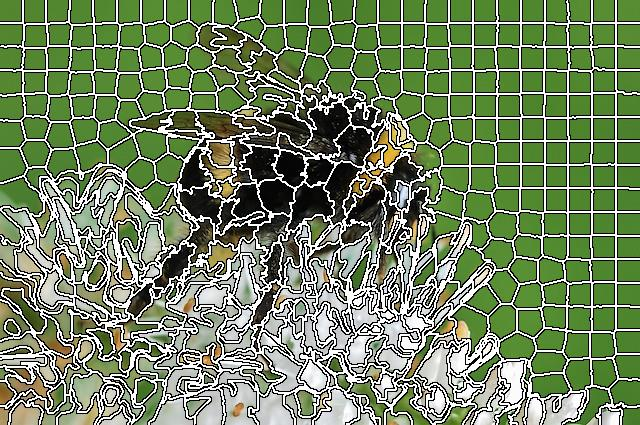展开全文• FindAroundLine.m是用来将超像素块画边界线的function函数 SLIC.m和FindAroundLine.m都不用运行，把他放在和RunMe同一个路径下就行 注意在运行RunMe.m脚本时，要将matlab的当前文件夹改为RunMe所在文件夹，里面的...
• SLIC超像素分割MATLAB代码夜间天空/云图像分割 本着可重复研究的精神，该存储库包含在手稿中产生结果所需的所有代码：S. Dev、FM Savoy、YH Lee 和 S. Winkler，夜间天空/云图像分割， Proc。 IEEE 国际图像处理会议...
• SLIC超像素分割算法MATLAB算法代码实现，超像素分割关键代码形式
• SLIC的matlab代码，可以更改自己的图片位置，生成结果，可以直接运行使用
• SLIC：simple linear iterative clustering,简单的线性迭代聚类，它使用k-means聚类来生成超像素，实现起来简单，运行效率还比较高，超像素对边界的保持效果页比较好，具体的与其他方法的对比结果在下方论文中有详细...

# 摘要：

SLIC：simple linear iterative clustering,简单的线性迭代聚类，它使用k-means聚类来生成超像素，实现起来简单，运行效率还比较高，超像素对边界的保持效果也比较好，具体的与其他方法的对比结果在下方论文中有详细记录。

### 文章目录

论文地址：https://infoscience.epfl.ch/record/177415/files/Superpixel_PAMI2011-2.pdf
代码地址：https://www.epfl.ch/labs/ivrl/research/slic-superpixels/#SLICO

# 1.原理

SLIC本质上说只需要一个聚类数k，这个聚类数k也就是超像素的个数，希望把图片分割成多少个超像素就设多少个聚类中心，在初始化时把聚类中心等间隔的放到图像上，设图像像素数为N，则在每个边长为 N / k \sqrt{N/k} 的方格内放置一个初始聚类中心， 为了避免把噪声像素和边缘当作聚类中心，会把该中心移动到周围3*3邻域内梯度最小的地方。
在用k-means做聚类时，采用的特征是Lab颜色空间的三个值加上坐标x，y，一共5个维度， x i . f e a t u r e = [ L , a , b , x , y ] T x_{i.feature} = [L,a,b,x,y]^T ,另外与常规的k均值不同的地方是，这里计算距离时只考虑聚类中心周围 2 N / k ∗ 2 N / k 2\sqrt{N/k} * 2\sqrt{N/k} 的邻域，因此计算量和一般的k均值聚类相比要小很多。搜索空间对比如下图， S = N / k S = \sqrt{N/k}聚类后计算每一类的平均特征，并把聚类中心的特征更新为平均特征。如果迭代超过10次或者前后两次聚类的差小于阈值则结束，否则继续聚类，更新聚类中心…。算法流程如下：# 2. 实现

## 2.1 初始化聚类中心

原文中按聚类数k来等间隔地初始化聚类中心，假设样本总数为N，聚类数为k，则每隔 N / k N/k 个样本放置一个聚类中心。在图片上等间隔地放置k个初始聚类中心，也就是把图片等分成边长为 N / k \sqrt{N/k} 的格子，在格子的固定位置放置初始聚类中心。另外为了避免初始的聚类中心落在物体边缘上，还要对每一个聚类中心都进行一下微调，具体就是计算初始聚类中心点周围邻域的梯度，把中心点移到梯度最小的点上。
这里实现的时候以初始超像素的边长len作为参数会比较直观，可以很简单的控制生成的超像素的大小，k和len的关系是 l e n = N / k len=\sqrt{N/k}
注：图片坐标系以左上角为原点，水平向右为x轴正方向，水平向下为y轴正方向(与opencv一致)，在访问图片数据矩阵时，一般用行和列的方式来描述，也就是row对应y，colum对应x。

• 等间隔放置聚类中心,
int initilizeCenters(cv::Mat &imageLAB, std::vector<center> &centers, int len)
{
if (imageLAB.empty())
{
std::cout << "In itilizeCenters:     image is empty!\n";
return -1;
}

uchar *ptr = NULL;
center cent;
int num = 0;
for (int i = 0; i < imageLAB.rows; i += len)
{
cent.y = i + len / 2;
if (cent.y >= imageLAB.rows) continue;
ptr = imageLAB.ptr<uchar>(cent.y);
for (int j = 0; j < imageLAB.cols; j += len)
{
cent.x = j + len / 2;
if ((cent.x >= imageLAB.cols)) continue;
cent.L = *(ptr + cent.x * 3);
cent.A = *(ptr + cent.x * 3 + 1);
cent.B = *(ptr + cent.x * 3 + 2);
cent.label = ++num;
centers.push_back(cent);
}
}
return 0;
}

• 把聚类中心移到到周围8邻域里梯度最小的地方,梯度用Sobel计算(不希望聚类中心落在边缘上所以才调整的)
int fituneCenter(cv::Mat &imageLAB, cv::Mat &sobelGradient, std::vector<center> &centers)
{

center cent;
uchar *imgPtr = imageLAB.ptr<uchar>(0);
for (int ck = 0; ck < centers.size(); ck++)
{
cent = centers[ck];
if (cent.x - 1 < 0 || cent.x + 1 >= sobelGradient.cols || cent.y - 1 < 0 || cent.y + 1 >= sobelGradient.rows)
{
continue;
}//end if
int tempx = 0, tempy = 0;
for (int m = -1; m < 2; m++)
{
for (int n = -1; n < 2; n++)
{
double gradient = pow(*(sobPtr + (cent.x + n) * 3), 2)
+ pow(*(sobPtr + (cent.x + n) * 3 + 1), 2)
+ pow(*(sobPtr + (cent.x + n) * 3 + 2), 2);
{
tempy = m;//row
tempx = n;//column
}//end if
}
}
cent.x += tempx;
cent.y += tempy;
imgPtr = imageLAB.ptr<uchar>(cent.y);
centers[ck].x = cent.x;
centers[ck].y = cent.y;
centers[ck].L = *(imgPtr + cent.x * 3);
centers[ck].A = *(imgPtr + cent.x * 3 + 1);
centers[ck].B = *(imgPtr + cent.x * 3 + 2);

}//end for
return 0;
}


## 2.2 聚类

对每一个聚类中心center_k，计算它到周围2len*2len区域的所有点的距离，如果新计算的距离比原来的距离更小，则把该点归入center_k这一类。
注意聚类的本质就是“物以类聚”，判断样本点和聚类中心的“近似”程度，可以从两个方面来考察，一种是距离测度：距离越近越相似，另一种是相似性测度，例如角度相似系数，相关系数，指数相似系数等。
距离的计算方式有很多种，比如：

• 欧拉距离 d ( x ⃗ , y ⃗ ) = ∣ ∣ x ⃗ − y ⃗ ∣ ∣ 2 2 d(\vec{x},\vec{y}) = ||\vec{x}-\vec{y}||_2^2
• 城区距离 d ( x ⃗ , y ⃗ ) = ∣ x ⃗ − y ⃗ ∣ d(\vec{x},\vec{y}) = |\vec{x}-\vec{y}|
• 切比雪夫距离 d ( x ⃗ , y ⃗ ) = m a x ∣ x i − y i ∣ , i 表 示 维 度 d(\vec{x},\vec{y}) = max|x_i-y_i|,i表示维度
• 马氏距离(Mahalanobis): d ( x ⃗ , y ⃗ ) = ( x ⃗ − y ⃗ ) T V − 1 ( x ⃗ − y ⃗ ) , V 表 示 样 本 总 体 的 协 方 差 矩 阵 ， V = 1 n − 1 Σ i = 1 n ( x i − x ⃗ ˉ ⃗ ) ( x i − x ⃗ ˉ ⃗ ) T , n 为 样 本 数 ， x i ⃗ 为 第 i 个 样 本 ( 列 向 量 ) ， x ⃗ ˉ 为 样 本 均 值 d(\vec{x},\vec{y}) = (\vec{x}-\vec{y})^TV^{-1}(\vec{x}-\vec{y}),V表示样本总体的协方差矩阵，V= \frac{1}{n-1}\Sigma_{i=1}^{n}(\vec{x_i - \bar{\vec{x}}})(\vec{x_i - \bar{\vec{x}}})^T,n为样本数， \vec{x_i}为第i个样本(列向量)， \bar{\vec{x}}为样本均值 ，它有一点比较好的性质就是与量纲无关（另外它还对坐标尺度、旋转、平移保持不变，从统计意义上去除了分量间的相关性），在这里分割超像素时，Lab颜色空间的距离往往会比空间距离大很多，用欧式距离时需要加一个权重参数来调节颜色距离和空间距离的比例。

要是以后有时间的话可以考虑一下都试一下这些距离聚类的效果。这里采用的是欧式距离，而且因为Lab颜色空间和图像xy坐标空间量纲不同，需要调整颜色空间距离和xy坐标距离的权重，论文中用下面的方式来计算距离
d c = ( l j − l i ) 2 + ( a j − a i ) 2 + ( b j − b i ) 2 d s = ( x j − x i ) 2 + ( y j − y i ) 2 D = d c 2 + ( d s S ) 2 m 2 \begin{aligned} d_{c}&=\sqrt{\left(l_{j}-l_{i}\right)^{2}+\left(a_{j}-a_{i}\right)^{2}+\left(b_{j}-b_{i}\right)^{2}} \\ d_{s} &=\sqrt{\left(x_{j}-x_{i}\right)^{2}+\left(y_{j}-y_{i}\right)^{2}}\\D&=\sqrt{d_{c}^{2}+\left(\frac{d_{s}}{S}\right)^{2} m^{2}} \end{aligned}
但是实际上在做超像素分割时我们更关心超像素的大小，而不是有多少个，虽然尺寸S和聚类数k有明确的对应关系，但是把k当输入参数不如直接用尺寸S来得直接，另外 d s 的 权 重 用 m 2 S 2 ds的权重用\frac{m^2}{S^2} 实际用起来有点麻烦，因为单独修改m或者s都会被另外一个参数调制，所以D的计算我改成了下面这样 D = d c 2 + m d s 2 \begin{aligned}D=\sqrt{d_{c}^{2}+md_{s}^{2} }\end{aligned}

int clustering(const cv::Mat &imageLAB, cv::Mat &DisMask, cv::Mat &labelMask,
std::vector<center> &centers, int len, int m)
{
if (imageLAB.empty())
{
std::cout << "clustering :the input image is empty!\n";
return -1;
}

double *disPtr = NULL;//disMask type: 64FC1
double *labelPtr = NULL;//labelMask type: 64FC1
const uchar *imgPtr = NULL;//imageLAB type: 8UC3

//disc = std::sqrt(pow(L - cL, 2)+pow(A - cA, 2)+pow(B - cB,2))
//diss = std::sqrt(pow(x-cx,2) + pow(y-cy,2));
//dis = sqrt(disc^2 + (diss/len)^2 * m^2)
double dis = 0, disc = 0, diss = 0;
//cluster center's cx, cy,cL,cA,cB;
int cx, cy, cL, cA, cB, clabel;
//imageLAB's  x, y, L,A,B
int x, y, L, A, B;

//注：这里的图像坐标以左上角为原点，水平向右为x正方向,水平向下为y正方向，与opencv保持一致
//      从矩阵行列角度看，i表示行，j表示列，即(i,j) = (y,x)
for (int ck = 0; ck < centers.size(); ++ck)
{
cx = centers[ck].x;
cy = centers[ck].y;
cL = centers[ck].L;
cA = centers[ck].A;
cB = centers[ck].B;
clabel = centers[ck].label;

for (int i = cy - len; i < cy + len; i++)
{
if (i < 0 | i >= imageLAB.rows) continue;
//pointer point to the ith row
imgPtr = imageLAB.ptr<uchar>(i);
for (int j = cx - len; j < cx + len; j++)
{
if (j < 0 | j >= imageLAB.cols) continue;
L = *(imgPtr + j * 3);
A = *(imgPtr + j * 3 + 1);
B = *(imgPtr + j * 3 + 2);

disc = std::sqrt(pow(L - cL, 2) + pow(A - cA, 2) + pow(B - cB, 2));
diss = std::sqrt(pow(j - cx, 2) + pow(i - cy, 2));
dis = sqrt(pow(disc, 2) + m * pow(diss, 2));

if (dis < *(disPtr + j))
{
*(disPtr + j) = dis;
*(labelPtr + j) = clabel;
}//end if
}//end for
}
}//end for (int ck = 0; ck < centers.size(); ++ck)

return 0;
}


## 2.3 更新聚类中心

对每一个聚类中心center_k，把所有属于这一类的点的特征加起来求平均，把这个平均特征赋给center_k。

int updateCenter(cv::Mat &imageLAB, cv::Mat &labelMask, std::vector<center> &centers, int len)
{
double *labelPtr = NULL;//labelMask type: 64FC1
const uchar *imgPtr = NULL;//imageLAB type: 8UC3
int cx, cy;

for (int ck = 0; ck < centers.size(); ++ck)
{
double sumx = 0, sumy = 0, sumL = 0, sumA = 0, sumB = 0, sumNum = 0;
cx = centers[ck].x;
cy = centers[ck].y;
for (int i = cy - len; i < cy + len; i++)
{
if (i < 0 | i >= imageLAB.rows) continue;
//pointer point to the ith row
imgPtr = imageLAB.ptr<uchar>(i);
for (int j = cx - len; j < cx + len; j++)
{
if (j < 0 | j >= imageLAB.cols) continue;

if (*(labelPtr + j) == centers[ck].label)
{
sumL += *(imgPtr + j * 3);
sumA += *(imgPtr + j * 3 + 1);
sumB += *(imgPtr + j * 3 + 2);
sumx += j;
sumy += i;
sumNum += 1;
}//end if
}
}
//update center
if (sumNum == 0) sumNum = 0.000000001;
centers[ck].x = sumx / sumNum;
centers[ck].y = sumy / sumNum;
centers[ck].L = sumL / sumNum;
centers[ck].A = sumA / sumNum;
centers[ck].B = sumB / sumNum;

}//end for

return 0;
}


## 2.4 显示超像素分割结果

方式一：把属于同一类的点的特征都替换成平均特征;

方式二：画出聚类边界;

# 3. 实测效果

• 左侧为原图，中间为在原图上画出超像素边界效果图，右侧为超像素图像效果# 4. 完整源码

//
//created by Mr. Peng. 2021\08\31
//

#include "opencv.hpp"

struct center
{
int x;//column
int y;//row
int L;
int A;
int B;
int label;
};

/
//input parameters:
//imageLAB:    the source image in Lab color space
//DisMask:       it save the shortest distance to the nearest center
//labelMask:   it save every pixel's label
//centers:       clustering center
//len:         the super pixls will be initialize to len*len
//m:           a parameter witch adjust the weights of the spacial and color space distance
//
//output:

std::vector<center> &centers, int len, int m)
{
if (imageLAB.empty())
{
std::cout << "clustering :the input image is empty!\n";
return -1;
}

double *disPtr = NULL;//disMask type: 64FC1
double *labelPtr = NULL;//labelMask type: 64FC1
const uchar *imgPtr = NULL;//imageLAB type: 8UC3

//disc = std::sqrt(pow(L - cL, 2)+pow(A - cA, 2)+pow(B - cB,2))
//diss = std::sqrt(pow(x-cx,2) + pow(y-cy,2));
//dis = sqrt(disc^2 + (diss/len)^2 * m^2)
double dis = 0, disc = 0, diss = 0;
//cluster center's cx, cy,cL,cA,cB;
int cx, cy, cL, cA, cB, clabel;
//imageLAB's  x, y, L,A,B
int x, y, L, A, B;

//注：这里的图像坐标以左上角为原点，水平向右为x正方向,水平向下为y正方向，与opencv保持一致
//      从矩阵行列角度看，i表示行，j表示列，即(i,j) = (y,x)
for (int ck = 0; ck < centers.size(); ++ck)
{
cx = centers[ck].x;
cy = centers[ck].y;
cL = centers[ck].L;
cA = centers[ck].A;
cB = centers[ck].B;
clabel = centers[ck].label;

for (int i = cy - len; i < cy + len; i++)
{
if (i < 0 | i >= imageLAB.rows) continue;
//pointer point to the ith row
imgPtr = imageLAB.ptr<uchar>(i);
for (int j = cx - len; j < cx + len; j++)
{
if (j < 0 | j >= imageLAB.cols) continue;
L = *(imgPtr + j * 3);
A = *(imgPtr + j * 3 + 1);
B = *(imgPtr + j * 3 + 2);

disc = std::sqrt(pow(L - cL, 2) + pow(A - cA, 2) + pow(B - cB, 2));
diss = std::sqrt(pow(j - cx, 2) + pow(i - cy, 2));
dis = sqrt(pow(disc, 2) + m * pow(diss, 2));

if (dis < *(disPtr + j))
{
*(disPtr + j) = dis;
*(labelPtr + j) = clabel;
}//end if
}//end for
}
}//end for (int ck = 0; ck < centers.size(); ++ck)

return 0;
}

/
//input parameters:
//imageLAB:    the source image in Lab color space
//labelMask:    it save every pixel's label
//centers:       clustering center
//len:         the super pixls will be initialize to len*len
//
//output:

int updateCenter(cv::Mat &imageLAB, cv::Mat &labelMask, std::vector<center> &centers, int len)
{
double *labelPtr = NULL;//labelMask type: 64FC1
const uchar *imgPtr = NULL;//imageLAB type: 8UC3
int cx, cy;

for (int ck = 0; ck < centers.size(); ++ck)
{
double sumx = 0, sumy = 0, sumL = 0, sumA = 0, sumB = 0, sumNum = 0;
cx = centers[ck].x;
cy = centers[ck].y;
for (int i = cy - len; i < cy + len; i++)
{
if (i < 0 | i >= imageLAB.rows) continue;
//pointer point to the ith row
imgPtr = imageLAB.ptr<uchar>(i);
for (int j = cx - len; j < cx + len; j++)
{
if (j < 0 | j >= imageLAB.cols) continue;

if (*(labelPtr + j) == centers[ck].label)
{
sumL += *(imgPtr + j * 3);
sumA += *(imgPtr + j * 3 + 1);
sumB += *(imgPtr + j * 3 + 2);
sumx += j;
sumy += i;
sumNum += 1;
}//end if
}
}
//update center
if (sumNum == 0) sumNum = 0.000000001;
centers[ck].x = sumx / sumNum;
centers[ck].y = sumy / sumNum;
centers[ck].L = sumL / sumNum;
centers[ck].A = sumA / sumNum;
centers[ck].B = sumB / sumNum;

}//end for

return 0;
}

int showSLICResult(const cv::Mat &image, cv::Mat &labelMask, std::vector<center> &centers, int len)
{
cv::Mat dst = image.clone();
cv::cvtColor(dst, dst, cv::COLOR_BGR2Lab);
double *labelPtr = NULL;//labelMask type: 32FC1
uchar *imgPtr = NULL;//image type: 8UC3

int cx, cy;
double sumx = 0, sumy = 0, sumL = 0, sumA = 0, sumB = 0, sumNum = 0.00000001;
for (int ck = 0; ck < centers.size(); ++ck)
{
cx = centers[ck].x;
cy = centers[ck].y;

for (int i = cy - len; i < cy + len; i++)
{
if (i < 0 | i >= image.rows) continue;
//pointer point to the ith row
imgPtr = dst.ptr<uchar>(i);
for (int j = cx - len; j < cx + len; j++)
{
if (j < 0 | j >= image.cols) continue;

if (*(labelPtr + j) == centers[ck].label)
{
*(imgPtr + j * 3) = centers[ck].L;
*(imgPtr + j * 3 + 1) = centers[ck].A;
*(imgPtr + j * 3 + 2) = centers[ck].B;
}//end if
}
}
}//end for

cv::cvtColor(dst, dst, cv::COLOR_Lab2BGR);
cv::namedWindow("showSLIC", 0);
cv::imshow("showSLIC", dst);
cv::waitKey(1);

return 0;
}

int showSLICResult2(const cv::Mat &image, cv::Mat &labelMask, std::vector<center> &centers, int len)
{
cv::Mat dst = image.clone();
//cv::cvtColor(dst, dst, cv::COLOR_Lab2BGR);
double *labelPtr = NULL;//labelMask type: 32FC1
double *labelPtr_nextRow = NULL;//labelMask type: 32FC1
uchar *imgPtr = NULL;//image type: 8UC3

for (int i = 0; i < labelMask.rows - 1; i++)
{
imgPtr = dst.ptr<uchar>(i);
for (int j = 0; j < labelMask.cols - 1; j++)
{
//if left pixel's label is different from the right's
if (*(labelPtr + j) != *(labelPtr + j + 1))
{
*(imgPtr + 3 * j) = 0;
*(imgPtr + 3 * j + 1) = 0;
*(imgPtr + 3 * j + 2) = 0;
}

//if the upper pixel's label is different from the bottom's
if (*(labelPtr_nextRow + j) != *(labelPtr + j))
{
*(imgPtr + 3 * j) = 0;
*(imgPtr + 3 * j + 1) = 0;
*(imgPtr + 3 * j + 2) = 0;
}
}
}

//show center
for (int ck = 0; ck < centers.size(); ck++)
{
imgPtr = dst.ptr<uchar>(centers[ck].y);
*(imgPtr + centers[ck].x * 3) = 100;
*(imgPtr + centers[ck].x * 3 + 1) = 100;
*(imgPtr + centers[ck].x * 3 + 1) = 10;
}

cv::namedWindow("showSLIC2", 0);
cv::imshow("showSLIC2", dst);
cv::waitKey(1);
return 0;
}

int initilizeCenters(cv::Mat &imageLAB, std::vector<center> &centers, int len)
{
if (imageLAB.empty())
{
std::cout << "In itilizeCenters:     image is empty!\n";
return -1;
}

uchar *ptr = NULL;
center cent;
int num = 0;
for (int i = 0; i < imageLAB.rows; i += len)
{
cent.y = i + len / 2;
if (cent.y >= imageLAB.rows) continue;
ptr = imageLAB.ptr<uchar>(cent.y);
for (int j = 0; j < imageLAB.cols; j += len)
{
cent.x = j + len / 2;
if ((cent.x >= imageLAB.cols)) continue;
cent.L = *(ptr + cent.x * 3);
cent.A = *(ptr + cent.x * 3 + 1);
cent.B = *(ptr + cent.x * 3 + 2);
cent.label = ++num;
centers.push_back(cent);
}
}
return 0;
}

//if the center locates in the edges, fitune it's location.
int fituneCenter(cv::Mat &imageLAB, cv::Mat &sobelGradient, std::vector<center> &centers)
{

center cent;
uchar *imgPtr = imageLAB.ptr<uchar>(0);
for (int ck = 0; ck < centers.size(); ck++)
{
cent = centers[ck];
if (cent.x - 1 < 0 || cent.x + 1 >= sobelGradient.cols || cent.y - 1 < 0 || cent.y + 1 >= sobelGradient.rows)
{
continue;
}//end if
int tempx = 0, tempy = 0;
for (int m = -1; m < 2; m++)
{
for (int n = -1; n < 2; n++)
{
double gradient = pow(*(sobPtr + (cent.x + n) * 3), 2)
+ pow(*(sobPtr + (cent.x + n) * 3 + 1), 2)
+ pow(*(sobPtr + (cent.x + n) * 3 + 2), 2);
{
tempy = m;//row
tempx = n;//column
}//end if
}
}
cent.x += tempx;
cent.y += tempy;
imgPtr = imageLAB.ptr<uchar>(cent.y);
centers[ck].x = cent.x;
centers[ck].y = cent.y;
centers[ck].L = *(imgPtr + cent.x * 3);
centers[ck].A = *(imgPtr + cent.x * 3 + 1);
centers[ck].B = *(imgPtr + cent.x * 3 + 2);

}//end for
return 0;
}

/
//input parameters:
//image:    the source image in RGB color space
//resultLabel:     it save every pixel's label
//len:         the super pixls will be initialize to len*len
//m:           a parameter witch adjust the weights of diss
//output:

int SLIC(cv::Mat &image, cv::Mat &resultLabel, std::vector<center> &centers, int len, int m)
{
if (image.empty())
{
std::cout << "in SLIC the input image is empty!\n";
return -1;

}

int MAXDIS = 999999;
int height, width;
height = image.rows;
width = image.cols;

//convert color
cv::Mat imageLAB;
cv::cvtColor(image, imageLAB, cv::COLOR_BGR2Lab);

cv::Sobel(imageLAB, sobelImagex, CV_64F, 0, 1, 3);
cv::Sobel(imageLAB, sobelImagey, CV_64F, 1, 0, 3);

//initiate
//std::vector<center> centers;
//disMask save the distance of the pixels to center;
//labelMask save the label of the pixels
cv::Mat labelMask = cv::Mat::zeros(cv::Size(width, height), CV_64FC1);

//initialize centers,  get centers
initilizeCenters(imageLAB, centers, len);
//if the center locates in the edges, fitune it's location

//update cluster 10 times
for (int time = 0; time < 10; time++)
{
//clustering
disMask = cv::Mat(height, width, CV_64FC1, cv::Scalar(MAXDIS));
//update
}

return 0;
}

int SLIC_Demo()
{

std::string imagePath = "K:\\deepImage\\plane.jpg";
cv::Mat dst;//save the shortest distance to the nearest centers
std::vector<center> centers;//clustering centers

int len = 25;//the scale of the superpixel ,len*len
int m = 10;//a parameter witch adjust the weights of spacial distance and the color space distance

cv::namedWindow("image", 1);
cv::imshow("image", image);

cv::waitKey(0);
return 0;
}

int main()
{
SLIC_Demo();
return 0;
}

展开全文c++ kmeans算法
• SLIC超像素分割MATLAB代码演示_SSAL_SDP 所提出的 SSAL-SDP 方法的演示，即基于超像素的半监督主动学习 (SSAL) 方法与密度峰值 (DP) 增强，用于高光谱图像分类。 这组文件包含用于基于超像素的半监督主动学习 (SSAL)...
• SLIC： simple linear ... 这是一个基于聚类算法的超像素分割，由LAB空间以及x、y像素坐标共5维空间来计算。不仅可以分割彩色图，也可以兼容分割灰度图，它还有一个优点就是可以人为的设置需要分割的超像素的数量。
• 目前只看到SLIC的简介和C++代码，有兄弟评论需要matlab版，这里给大家找来了，记得给好评喔
• SLIC超像素分割详解（一）：简介 超像素概念是2003年Xiaofeng Ren提出和发展起来的图像分割技术，是指具有相似纹理、颜色、亮度等特征的相邻像素构成的有一定视觉意义的不规则像素块。它利用像素之间特征的相似性将...

# SLIC超像素分割详解（一）：简介

超像素概念是2003年Xiaofeng Ren提出和发展起来的图像分割技术，是指具有相似纹理、颜色、亮度等特征的相邻像素构成的有一定视觉意义的不规则像素块。它利用像素之间特征的相似性将像素分组,用少量的超像素代替大量的像素来表达图片特征,很大程度上降低了图像后处理的复杂度，所以通常作为分割算法的预处理步骤。已经广泛用于图像分割、姿势估计、目标跟踪、目标识别等计算机视觉应用。几种常见的超像素分割方法及其效果对比如下：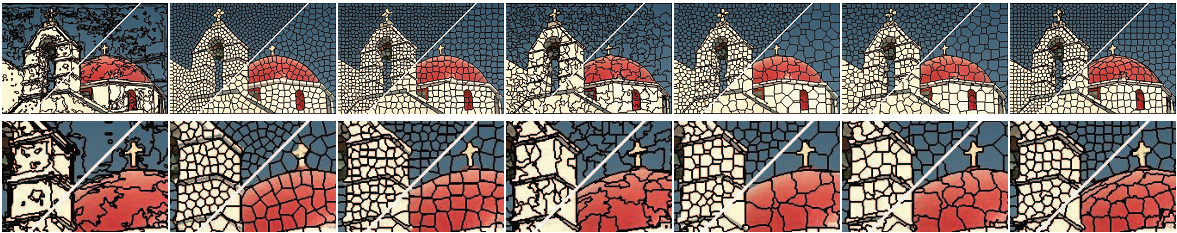Graph-based           NCut            Turbopixel          Quick-shift        Graph-cut a        Graph-cut b         SLIC

这里主要介绍的是SLIC（simple linear iterativeclustering），即简单的线性迭代聚类。它是2010年提出的一种思想简单、实现方便的算法，将彩色图像转化为CIELAB颜色空间和XY坐标下的5维特征向量，然后对5维特征向量构造距离度量标准，对图像像素进行局部聚类的过程。SLIC算法能生成紧凑、近似均匀的超像素，在运算速度，物体轮廓保持、超像素形状方面具有较高的综合评价，比较符合人们期望的分割效果。

SLIC主要优点总结如下：1）生成的超像素如同细胞一般紧凑整齐，邻域特征比较容易表达。这样基于像素的方法可以比较容易的改造为基于超像素的方法。2）不仅可以分割彩色图，也可以兼容分割灰度图。3）需要设置的参数非常少，默认情况下只需要设置一个预分割的超像素的数量。4）相比其他的超像素分割方法，SLIC在运行速度、生成超像素的紧凑度、轮廓保持方面都比较理想。

在介绍SLIC之前，插播一下Lab颜色空间的介绍。Lab色彩模型是由亮度（L）和有关色彩的a, b三个要素组成。L表示亮度（Luminosity），L的值域由0（黑色）到100（白色）。a表示从洋红色至绿色的范围（a为负值指示绿色而正值指示品红），b表示从黄色至蓝色的范围（b为负值指示蓝色而正值指示黄色）。Lab颜色空间的优点：1）不像RGBCMYK色彩空间，Lab 颜色被设计来接近人类生理视觉。它致力于感知均匀性，它的 L 分量密切匹配人类亮度感知。因此可以被用来通过修改 a 和 b 分量的输出色阶来做精确的颜色平衡，或使用 L 分量来调整亮度对比。这些变换在 RGB 或 CMYK 中是困难或不可能的。2）因为 Lab 描述的是颜色的显示方式，而不是设备（如显示器、打印机或数码相机）生成颜色所需的特定色料的数量，所以 Lab 被视为与设备无关的颜色模型。3）色域宽阔。它不仅包含了RGB，CMYK的所有色域，还能表现它们不能表现的色彩。人的肉眼能感知的色彩，都能通过Lab模型表现出来。另外，Lab色彩模型的绝妙之处还在于它弥补了RGB色彩模型色彩分布不均的不足，因为RGB模型在蓝色到绿色之间的过渡色彩过多，而在绿色到红色之间又缺少黄色和其他色彩。如果我们想在数字图形的处理中保留尽量宽阔的色域和丰富的色彩，最好选择Lab。

下面描述一下SLIC具体实现的步骤：

1.  初始化种子点（聚类中心）：按照设定的超像素个数，在图像内均匀的分配种子点。假设图片总共有 N 个像素点，预分割为 K 个相同尺寸的超像素，那么每个超像素的大小为N/ K ，则相邻种子点的距离（步长）近似为S=sqrt(N/K)。

2.  在种子点的n*n邻域内重新选择种子点（一般取n=3）。具体方法为：计算该邻域内所有像素点的梯度值，将种子点移到该邻域内梯度最小的地方。这样做的目的是为了避免种子点落在梯度较大的轮廓边界上，以免影响后续聚类效果。

3.  在每个种子点周围的邻域内为每个像素点分配类标签（即属于哪个聚类中心）。和标准的k-means在整张图中搜索不同，SLIC的搜索范围限制为2S*2S，可以加速算法收敛，如下图。在此注意一点：期望的超像素尺寸为S*S，但是搜索的范围是2S*2S。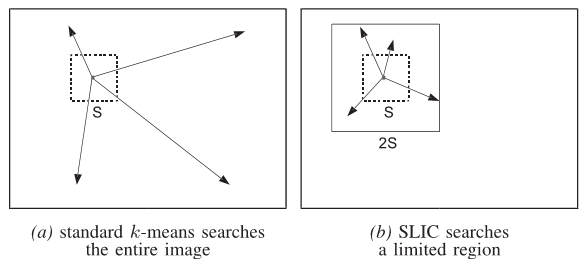4.  距离度量。包括颜色距离和空间距离。对于每个搜索到的像素点，分别计算它和该种子点的距离。距离计算方法如下：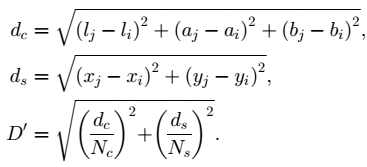其中，dc代表颜色距离，ds代表空间距离，Ns是类内最大空间距离，定义为Ns=S=sqrt(N/K)，适用于每个聚类。最大的颜色距离Nc既随图片不同而不同，也随聚类不同而不同，所以我们取一个固定常数m（取值范围[1,40],一般取10）代替。最终的距离度量D'如下：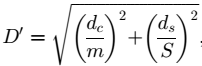由于每个像素点都会被多个种子点搜索到，所以每个像素点都会有一个与周围种子点的距离，取最小值对应的种子点作为该像素点的聚类中心。

5.  迭代优化。理论上上述步骤不断迭代直到误差收敛（可以理解为每个像素点聚类中心不再发生变化为止），实践发现10次迭代对绝大部分图片都可以得到较理想效果，所以一般迭代次数取10。

6.  增强连通性。经过上述迭代优化可能出现以下瑕疵：出现多连通情况、超像素尺寸过小，单个超像素被切割成多个不连续超像素等，这些情况可以通过增强连通性解决。主要思路是：新建一张标记表，表内元素均为-1，按照“Z”型走向（从左到右，从上到下顺序）将不连续的超像素、尺寸过小超像素重新分配给邻近的超像素，遍历过的像素点分配给相应的标签，直到所有点遍历完毕为止。

参考文献：

  Achanta,Radhakrishna, et al. Slic superpixels. No. EPFL REPORT 149300. 2010.

 Achanta,Radhakrishna, et al. "SLIC superpixels compared to state-of-the-artsuperpixel methods." Pattern Analysis and Machine Intelligence, IEEETransactions on 34.11 (2012): 2274-2282.

# SLIC超像素分割详解（二）：关键代码分析

网站http://ivrl.epfl.ch/research/superpixels给出了SLIC的代码。对于其中C++代码的几个关键函数实现细节解释一下，方便初学者理解。具体如下：

1、设定期望分割的超像素数目，打开图片。将彩色RGB图片转换为LAB空间及x、y像素坐标共5维空间。

2、DetectLabEdges。求图片中所有点的梯度=dx+dy.其中

dx=(l(x-1)-l(x+1))*(l(x-1)-l(x+1))+(a(x-1)-a(x+1))*(a(x-1)-a(x+1))+(b(x-1)-b(x+1))*(b(x-1)-b(x+1));

dy=(l(y-1)-l(y+1))*(l(y-1)-l(y+1))+(a(y-1)-a(y+1))*(a(y-1)-a(y+1))+(b(y-1)-b(y+1))*(b(y-1)-b(y+1));

3、GetLABXYSeeds_ForGivenK。给定了要分割的超像素总数K，根据LABXY信息获得种子点。

1)   超像素的种子点间步长Step=sqrt(N/K)。初始化种子点。按照步长均匀播撒种子点，初始化后种子点是均匀分布的（图1中的红色点）。

2)   PerturbSeeds。扰乱种子点。在每个种子点的3*3邻域内，计算该种子点的8个邻域内像素点的Lab颜色梯度(同上述步骤2)，分别与初始种子点梯度进行比较，取梯度值最小（最“平坦”）的点，并记录其LABXY信息作为新的种子点（图1中绿色点为扰乱后的新种子点）。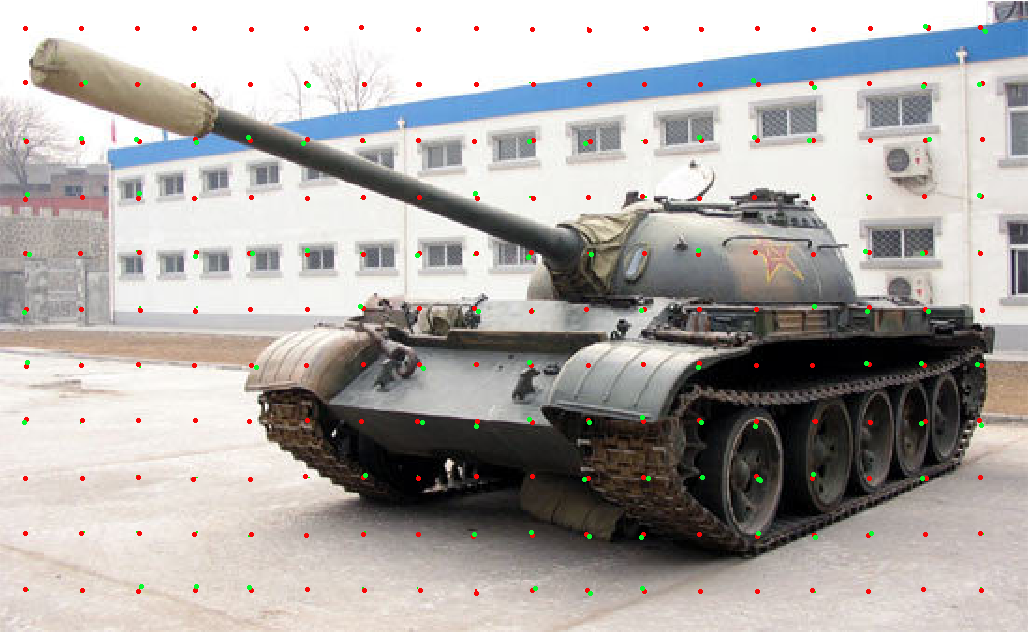图1：扰乱种子点图示

4、超像素的步长Step=sqrt(N/K)+2。加了一个小偏置2是为了避免Step太小，造成超像素太密集的情况。

5、PerformSuperpixelSegmentation_VariableSandM。对于每个超像素,最大的颜色距离M取值范围[1,40],一般取10。最大空间距离取步长为Step。

1)   搜索范围2step* 2step，即设置offset=step。 在步长较短时（step<10）可以扩展offset=step*1.5作为搜索范围。

2)  初始化distlab、distxy、distvec为无穷大。maxlab初始化为10*10，maxxy初始化为step*step。distlab代表某点与种子点的lab颜色空间距离，计算如下：distlab(i)=(l-kseedsl(n))*(l-kseedsl(n))+(a-kseedsa(n))*(a-kseedsa(n))+(b-kseedsb(n))*(b-kseedsb(n));distxy代表某点与种子点的空间坐标距离，计算如下：distxy(i)=(x-kseedsx(n))*(x-kseedsx(n))+(y-kseedsy(n))*(y-kseedsy(n));dist代表某点与种子点的综合距离（归一化的颜色距离+空间距离），计算如下：dist=distlab/( maxlab)+ distxy/(maxxy);在此提醒一下：如果将C++程序转为matlab代码时特别要注意数据类型。uint16类型变量减去double类型变量的结果是uint16类型，所以如果后者值大于前者，结果就为0。此处容易出错，需要强制类型转换。

3)   计算搜索区域内每个点离种子点的距离dist，并将搜索区域内每个点离种子点的距离保存在distvec中。因为某点可能位于多个种子点的搜索区域，所以最后保存的是离相邻种子点最近的距离，并将该像素标号为最近种子点相同的标号。同一个超像素内所有像素的标号相同

4)   计算每个新超像素内所有像素的labxy均值和坐标重心。将坐标重心作为该超像素的新种子点位置。

5)   上述步骤2)到4)重复迭代10次。

6、EnforceLabelConnectivity。该函数主要有几个作用：保证同一个超像素都是单连通区域；去掉尺寸过小的超像素；避免单个超像素被切割的情况。

1)   先计算超像素理想面积大小：SUPSZ = sz/K = N/K;

2)   有两种标号向量：上一步骤中得到的旧标号向量labels(即步骤5中得到的klabels)，但其存在多连通，过小超像素等问题，需要优化。新标号向量nlabels，初始化值全为-1。

3)   首先选择每个超像素的起始点（左上角的第一个点），起始点判断条件：a) 按照从左到右，从上到下的“Z”型顺序查找。b)该点在新标号向量nlabels中未被标记过（值为-1）。将其坐标保存在xvec,yvec中。

5)   扩展当前超像素。首先选择起始点作为当前操作的中心点，然后对其四邻域进行判断是否属于该超像素成员。判断条件：a) 该点在新标号向量nlabels中未被标记过（值为-1）；b)该点n和当前操作中心点c在旧标号向量中标号一致，即labels(n)= labels(c)，可以理解为原先就是属于同一个超像素的成员。如果判断是超像素的新成员，那么把该新成员作为新的操作中心点，循环直到找不到新成员为止。

7)   迭代上述步骤3）到6）直到整张图片遍历结束。

7、绘制分割结果，退出窗口。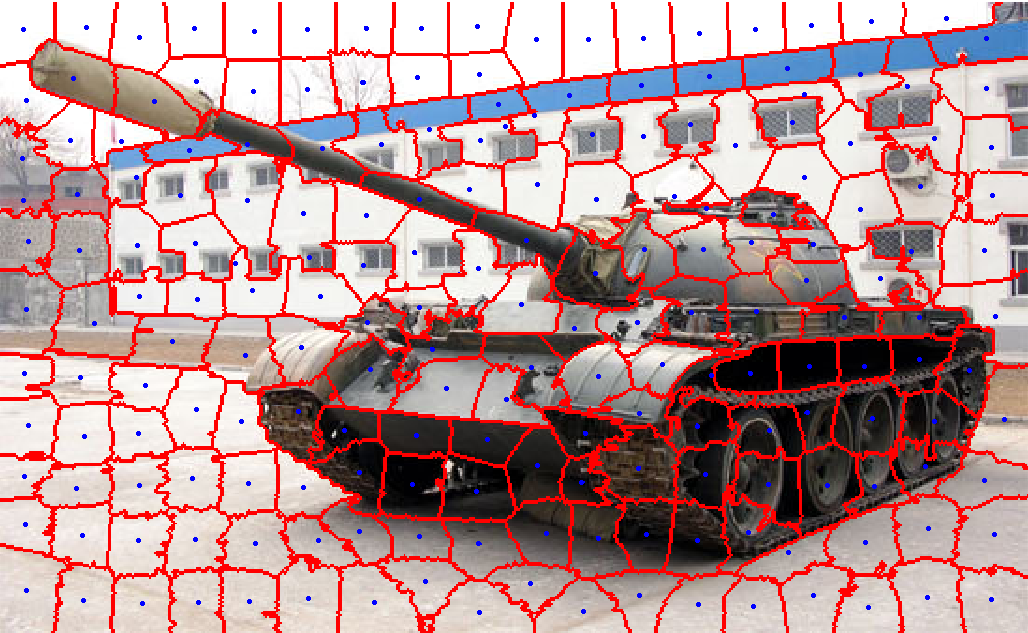图2：SLIC超像素分割结果，蓝色的点表示最终超像素的种子点。

# SLIC 超像素分割详解（三）：应用

看过上面的介绍后，我们应该思考一下：分割好的超像素有什么用？怎么用？用到哪里？

首先，超像素可以用来做跟踪，可以参考卢湖川课题组发表在IEEE TIP上的《Robust superpixeltracking》；其次，可以做标签分类，参考09年ICCV的《Class segmentation andobject localization with superpixel neighborhoods》，这篇文章对后来的文章还是有很大的指导意义的；还有做超像素级词袋（superpixel-basedbag-of-words）的，参考13年CVPR《Improving an objectdetector and extracting regions using superpixels》，作者把样本中超像素级的特征通过k-means聚类为超像素级词袋，最后又结合了SVM对难分类样本进一步分类，该文章的算法框图如下；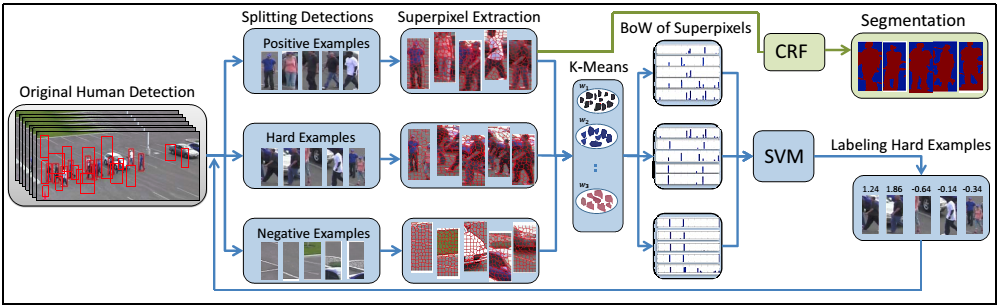超像素级词袋

在视频前景分割中用的也挺多，因为相比像素，超像素处理速度会快几十倍、几百倍甚至更高，这对实时性要求较高的视频分割比较重要，还有最近提出的supervoxel概念（可以认为是三维的superpixel），文章可以参考《Video object segmentationwith shape cue based on spatiotemporal superpixel neighbourhood》和《Supervoxel-consistentforeground propagation in video》。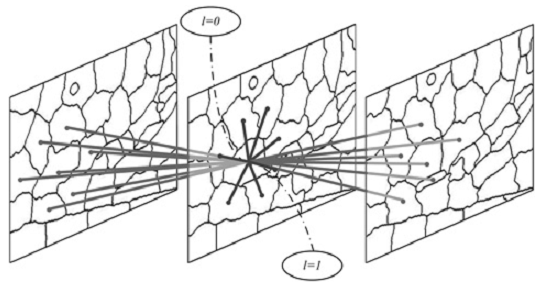Supervoxel (3D superpixel)示例

除了上述介绍的之外，超像素还可以用于骨架提取、人体姿态估计（参考文章《Guiding ModelSearch Using Segmentation》）、医学图像分割等方面。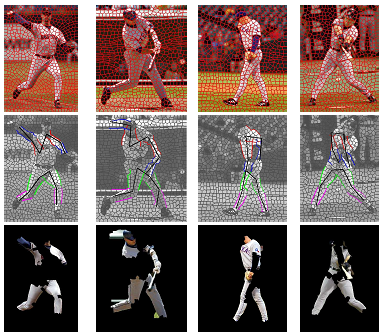骨架提取示例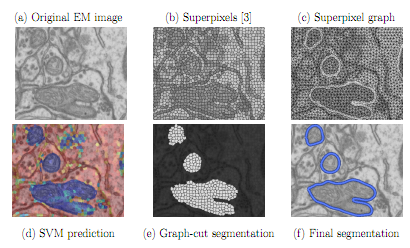医学图像分割

其实，传统的像素级处理都可以考虑改造成超像素级处理，所以如果你想用的话，超像素就可以应用到计算机视觉这个大领域的方方面面。举一个具体的例子进行分析：下图中每个红色闭环轮廓内都是一个超像素，像素级的光流改造（例如求均值）为超像素级的光流矢量用绿色的箭头表示。这样一张300万像素的图片可以用300个（给定分割数目K=300）超像素来表示。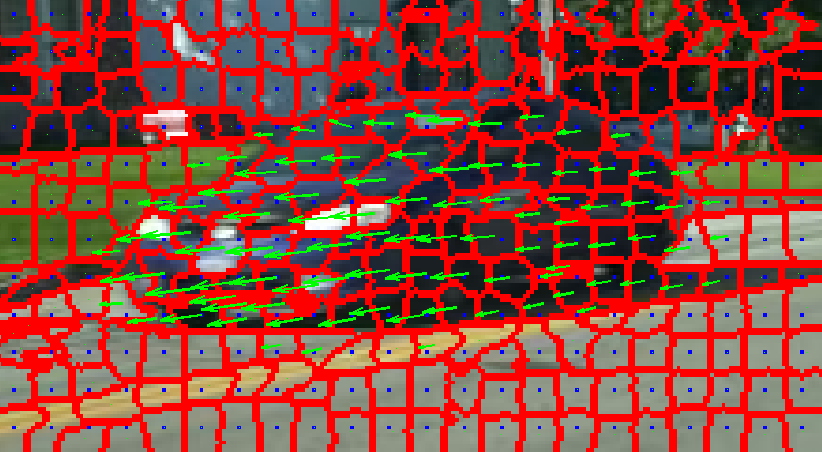下面以一篇文章《Improving video foreground segmentation with an object-like pool》下载论文为例具体分析一下超像素的用法。该文章目标是在无监督条件下对序列图像中运动目标进行精细的分割。算法流程如下：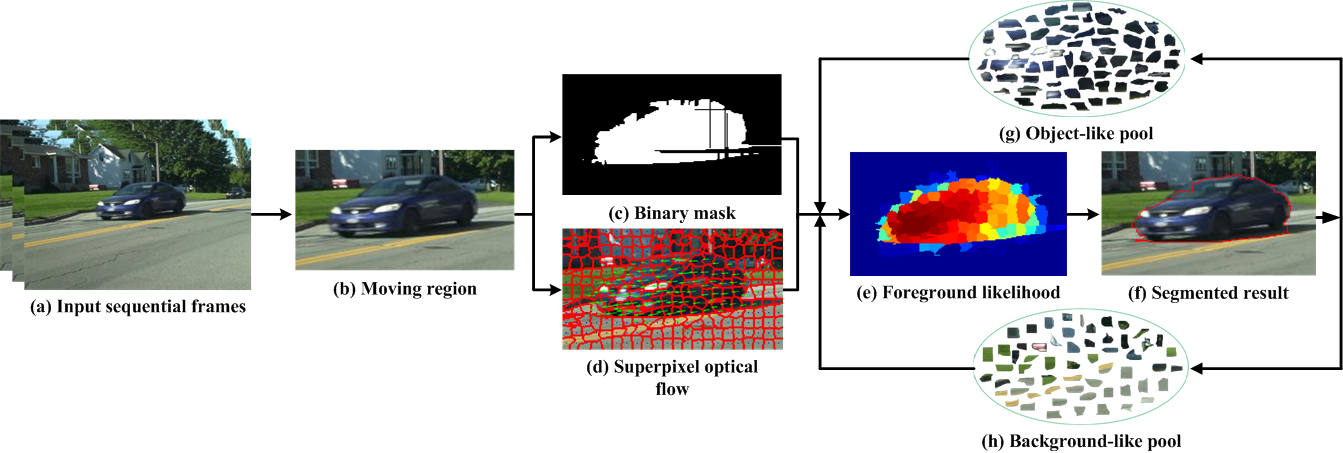算法流程图

2.  Binarymask特征通过2012年CVPRW的论文《Improving foreground segmentationswith probabilistic superpixel Markov random fields》中的方法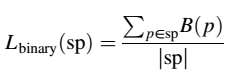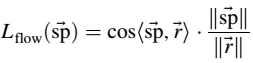Optical flow像素级特征改造稍微复杂一点，因为每个像素点是一个二维矢量，作者首先将平均的光流矢量作为该超像素的光流矢量，然后将所有超像素级的光流矢量均值作为参考矢量，再计算每个超像素矢量和参考矢量的余弦相似度作为该超像素在optical flow特征下属于前景/背景的概率。

3.  分割问题其实就是对每个超像素标记的问题，作者用conditional random field（条件随机场）来对超像素的分布进行建模，上述两种超像素级的特征都需要归一化到[0,1]并加权融合，结果作为unary potential（一元势函数，描述自身属于前景/背景概率）。Pairwise potential（二元势函数，描述邻域关系）的表达参照了像素级的做法：以种子点的四邻域作为中心超像素的邻域。在这里就体现出SLIC方法的优点了，分割结果比较紧凑而且大小相差不大，所以邻域关系相对于其他的超像素分割方法依然保持的比较好。

4.  Object-likepool和background-like pool是作者引入的概念，是以上一次分割的结果作为先验条件指导当前分割，其保留的也是超像素级的信息（颜色、光流、位置）。然后利用公式（11）计算出了一个新的归一化特征foreground likelihood，把它融入上述conditional random field模型。

下面讨论一下如何设置待分割超像素数目？使用超像素对图像进行分割时，设置的超像素数目K比较重要：如果K比较小，每个超像素尺寸会比较大，这样超像素对边界的保持就会变差，如果K比较大，每个超像素的尺寸会比较小，那么会出现类似“过拟合”现象，超像素的形状会变得非常不规则，邻域关系很难保持，而且数目也比较多。如下图：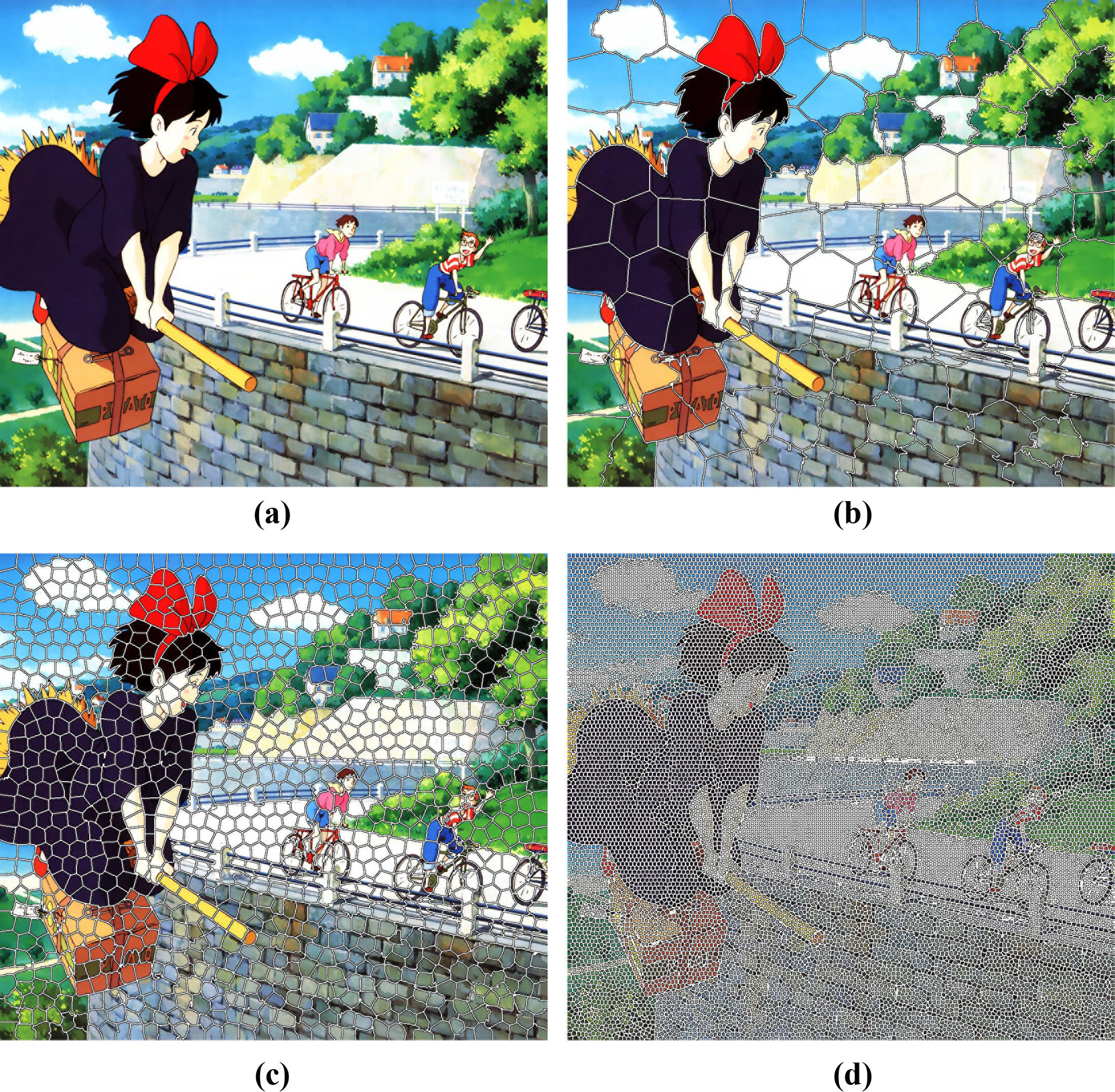不同尺寸的超像素分割结果对比。(a)原图，超像素尺寸：(b)100X100,(c)30X30,(d)8X8

实际上具体分割数目K和具体应用有关，比如如果对上图做主要人物（左边的小魔女）分割的话，100X100大小的超像素就够了，但是如果对两个骑自行的人物也进行分割的话，需要使用30X30的尺寸，但是此时较小人物的分割精度不高，如果有更高要求，则需要使用8X8甚至更小的尺寸。另外，由于上图是动画，所以细节和纹理并不是很多，现实场景下的图片纹理会复杂的多，尺寸也需要根据不同场合选择。

展开全文• 简单，可运行，代码只有几行，直接调用函数，图片都有。
• 之前做SLIC分割的过程中在网上找到的代码都只能得到标注分割线的图但不能得到分割后的超像素块，所以写了一个能够保存分割后的超像素块的python程序。 进行SLIC分割部分： import skimage from skimage....python slice 计算机视觉
• 一、SLIC概念 Python实现超像素分割 图像分割：Python的SLIC超像素分割 二、SLIC库的介绍 python 自带slic代码分析 ...SLIC超像素分割并保存分割得到的超像素块，python代码 超像素分割, 并获取每一个分区 ...算法 python 机器学习
• 最近在看显著性检测，发现很多算法的基础是超像素分割，而正在看的Saliency Optimization from Robust Background Detection算法的预处理是SLIC算法，于是便找了SLIC算法的论文进行学习，在学习过程中也顺便翻译了...
• SLIC超像素分割：将彩色图像转化为CIELAB颜色空间和XY坐标下的5维特征向量，然后对5维特征向量构造距离度量标准，对图像像素进行局部聚类的过程。
• slic超像素分割方法源代码。c++实现，简洁明了superpixel
• 介绍SLIC超像素分割算法，给出其与openCV的接口，代码用VS2012和openCV2.4.9测试可运行。opencv
• SLIC超像素分割的算法介绍和源码分析 源码分析：作者上传的并不是纯粹的matlab代码，而是采用c写的生成的mex文件。 使用前提：我的电脑安装Matlab 2016ra和Vs2015 首先在下载源码的文件夹下面打开matlab，要写实现c...
• 代码网址：http://ivrl.epfl.ch/research/superpixels#SLICO PS：上面的链接失效了，我搞了个代码托管，附链接：...下载了SLIC超像素分割的MATL...
• MATLAB 语言编写的SLIC超像素分割算法，代码块均有注释，运行速度快，效率好
• ## SLIC超像素分割算法

万次阅读 多人点赞 2017-09-22 18:17:13
超像素概念是2003年Xiaofeng Ren提出和发展起来的图像分割技术，是指具有相似纹理、颜色、亮度等特征的相邻像素构成的有一定视觉意义的不规则像素块。它利用像素之间特征的相似性将像素分组...几种常见的超像素分割方法
• 基于SLIC方法的超像素分割算法代码，纯MATLAB编写。
• 使用超像素的进行图像分割的matlab代码, 若体验良好可以给个好评
• 题目如下： 简单解法（HSV 直方图阈值）如下： ...这一节先讲SLIC超像素算法，下一节讲在超像素基础上用Kmeans分类进行分割，参考博客如下： https://www.jianshu.com/p/d0ef931b3ddf https://blog.csdn.net/duyue...opencv c++...